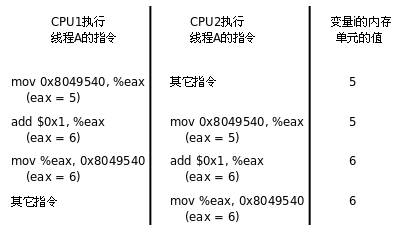# Linux线程同步-----互斥量(Mutex)#include <pthread.h>
pthread_mutex_t mutex = PTHREAD_MUTEX_INITIALIZER;

#include <pthread.h>
int pthread_mutex_unlock(pthread_mutex_t *mutex);//成功返回0，失败返回错误号

Mutex 锁的原理

lock:
if(mutex > 0)
{
mutex = 0;
return 0;
}
else
{
//suspend;
goto lock;
}
unlock:
mutex = 1;
唤醒其它因等待Mutex 而挂起的线程；
return 0;

lock:
movb $0, %al; xchgb %a1, mutex;//如果mutex 为0（被占用状态）那么交换后al 得到的是占用状态，原锁mutex 值不变（继续被占用）。 if(al > 0){ return 0; }else{ //suspend; goto lock; } unlock: movb$1, mutex;
唤醒其它因等待Mutex 而挂起的线程；
return 0;


Mutex小结

Mutex使用示例
#include <stdio.h>
#include <stdlib.h>
#include <string.h>
#include <unistd.h>
#include <sys/types.h>

int seq = 0;

int seq1 = 0;

void *thr_func_without_lock(void *arg)
{
printf("The sequence(without lock) is %d ...\r\n", ++seq1);
}

void *thr_func_with_lock(void *arg)
{
printf("The sequence is %d ...\r\n", ++seq);

return ((void *)0);
}

void demo()
{
int i= 0;
for (i = 0 ; i < 10000; ++i)
{
}
}

void lock_demo()
{
int i = 0;
for (i = 0 ; i < 10000; ++i)
{
}
}

int main(int argc, char **argv)
{
demo();
printf("\r\n");
lock_demo();
return 0;
}